##### Geometry For Dummies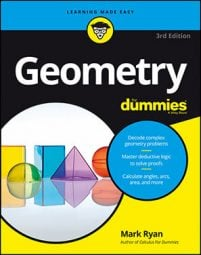A trapezoid is a quadrilateral (a shape with four sides) with exactly one pair of parallel sides (the parallel sides are called bases). The following figure shows a trapezoid to the left, and an isosceles trapezoid on the right.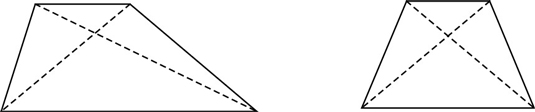• The properties of the trapezoid are as follows:

• The bases are parallel by definition.

• Each lower base angle is supplementary to the upper base angle on the same side.

• The properties of the isosceles trapezoid are as follows:

• The properties of a trapezoid apply by definition (parallel bases).

• The legs are congruent by definition.

• The lower base angles are congruent.

• The upper base angles are congruent.

• Any lower base angle is supplementary to any upper base angle.

• The diagonals are congruent.

The supplementary angles might be the hardest property to spot in the diagrams above. Because of the parallel sides, consecutive angles are same-side interior angles and are thus supplementary. (All the special quadrilaterals except the kite, by the way, contain consecutive supplementary angles.)

Here’s an isosceles trapezoid proof for you: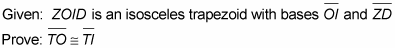Statement 1: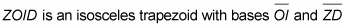Reason for statement 1: Given.

Statement 2: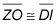Reason for statement 2: The legs of an isosceles trapezoid are congruent.

Statement 3: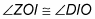Reason for statement 3: The upper base angles of an isosceles trapezoid are congruent.

Statement 4: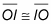Reason for statement 4: Reflexive Property.

Statement 5: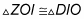Reason for statement 5: Side-Angle-Side, or SAS (2, 3, 4)

Statement 6: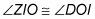Reason for statement 6: CPCTC (Corresponding Parts of Congruent Triangles are Congruent).

Statement 7: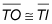Reason for statement 7: If angles are congruent, then so are sides.